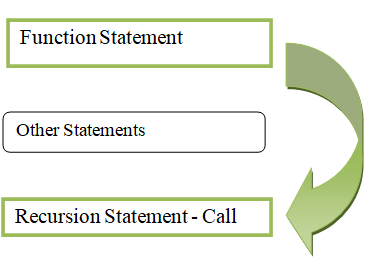# Understanding Recursive Functions with Python

• Last Updated : 15 Jul, 2021

Recursion is characterized as the process of describing something in terms of itself; in other words, it is the process of naming the function by itself. Recursion is the mechanism of a function calling itself directly or implicitly, and the resulting function is known as a Recursive function.

• Code reusability
• Easily understandable
• Time complexity sometimes low
• Less number of code

• Causes overflow if condition exceeds
• More memory
• It is difficult to understand
• It is difficult to debug
• Terminating conditions in Recursion is a must

Syntax:

def function_name():

……………..

|(recursive call)

………………….

function_name()Recursion calls the function which is already called and will call many times till the condition will become false. After that, it will return the value

### Applications:

• Tower of Hanoi
• Stack implementation
• Fibonacci implementation
• Round Robin problem in Operating System.

Apart from the above applications below are some examples that depict how to use recursive functions in a program.

Example 1:

Python program to print Fibonacci series up to given terms.

Fibonacci:

Number=sum of two previous numbers

0,1

0+1=1

0+1=2

2+1=3

3+2=5

5+3=8

So the Fibonacci numbers are 0,1,1,2,3,5,8…….

In this program, the values are defined in till_range variables and then we are passing that variable into an argument, then calling the recursive function.

`recursive_function(a-1) + recursive_function(a-2)`

Implementation:

## Python3

 `# Recursive function``def` `recursive_function(a):``  ` `    ``# Check number``    ``if` `a <``=` `1``:``        ``return` `a``    ``else``:``        ` `        ``# Get next term``        ``return``(recursive_function(a``-``1``) ``+` `recursive_function(a``-``2``))` `    ` `# Display first fibonacci number``print``(``"\nFibonacci series upto 1 number:"``)``for` `i ``in` `range``(``1``):``    ``print``(recursive_function(i),``          ``end``=``" "``)   ``    ` `# Display first 5 fibonacci numbers``print``(``"\nFibonacci series upto 5 numbers:"``)``for` `i ``in` `range``(``5``):``    ``print``(recursive_function(i),``          ``end``=``" "``)``    ` `# Display first 10 fibonacci numbers``print``(``"\nFibonacci series upto 10 numbers:"``)``for` `i ``in` `range``(``10``):``    ``print``(recursive_function(i),``          ``end``=``" "``)   `

Output:

```Fibonacci series upto 1 number:
0
Fibonacci series upto 5 numbers:
0 1 1 2 3
Fibonacci series upto 10 numbers:
0 1 1 2 3 5 8 13 21 34 ```

Example 2:

Python program to find factorial of a number.

Factorial

Number=Number*(Number-1)*(Number-2)…*(Number-(Number+1))

Factorial of 3= 3*2*1

Factorial of 5= 5*4*3*2*1

Factorial of 7 = 7*6*5*4*3*2*1

In this python program, we are going to return the factorial of an integer. We are passing the variable n value to an argument, then we are calling the function.

`a* recursion_factorial(a-1)`

Implementation:

## Python3

 `# recursive function``def` `recursion_factorial(a):``  ` `    ``# check number``    ``if` `a ``=``=` `1``:``        ``return` `1``    ``else``:``        ` `        ``# multiply with next number``        ``return` `(a ``*` `recursion_factorial(a``-``1``))`  `# factorial of 7``n ``=` `7``print``(``"Factorial of"``, n, ``"is"``, recursion_factorial(n))` `# factorial of 2``n ``=` `2``print``(``"Factorial of"``, n, ``"is"``, recursion_factorial(n))` `# factorial of 4``n ``=` `4``print``(``"Factorial of"``, n, ``"is"``, recursion_factorial(n))` `# factorial of 9``n ``=` `9``print``(``"Factorial of"``, n, ``"is"``, recursion_factorial(n))` `# factorial of 10``n ``=` `10``print``(``"Factorial of"``, n, ``"is"``, recursion_factorial(n))`

Output:

```Factorial of 7 is 5040
Factorial of 2 is 2
Factorial of 4 is 24
Factorial of 9 is 362880
Factorial of 10 is 3628800```

My Personal Notes arrow_drop_up